Author: Oscar Cronquist Article last updated on September 29, 2022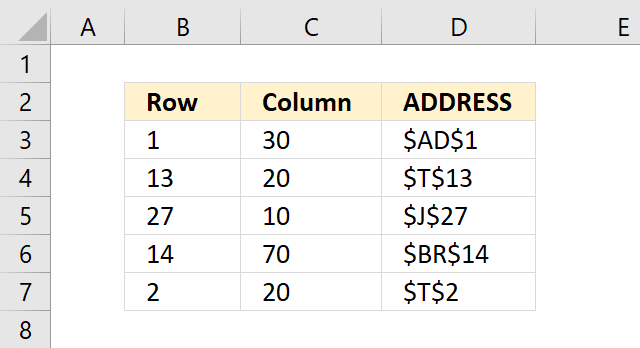The ADDRESS function returns the address of a specific cell, you need to provide a row and column number.

## 2. Arguments

 row_num Required. A number representing the row number. column_num Required. A number representing the column number. [abs_num] Optional. Lets you choose the type of reference to return. 1 - Absolute. 2 - Absolute row, relative column. 3 - Absolute column, relative row. 4 - Relative. [a1] Optional. Lets you choose reference style. A1 reference style is the default setting you are probably used to. The R1C1 reference style has both columns and rows are labeled numerically. TRUE - A1-style reference. FALSE - R1C1-style reference. [sheet_text] Optional. A text value that specifies the name of the worksheet to be used as the external reference. =ADDRESS(1,1,,,"Sheet2") returns Sheet2!\$A\$1. If omitted the function refers to a cell on the current sheet.Formula in cell D3:

### 3.1 Explaining formula

#### Step 1 - ADDRESS Function

The ADDRESS function calculates the address of a specific cell based on a row and column number.

#### Step 2 - Populate arguments

row_num - B3

column_num - C3

[abs_num], - optional

[a1] - optional

[sheet_text]) - optional

#### Step 3 - Evaluate ADDRESS function

and returns "\$AD\$1". This is a string, not a cell reference.

## 4. ADDRESS function not working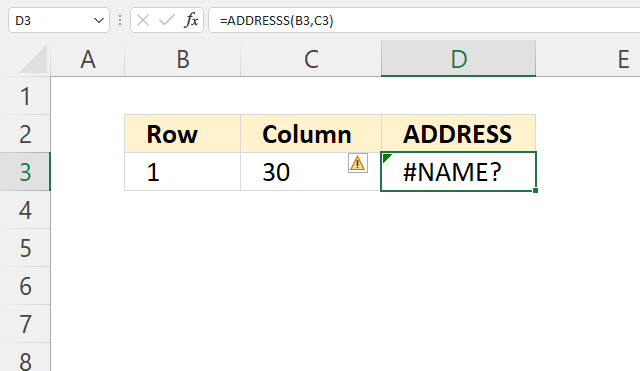Make sure your spelling is correct, the image above shows a #NAME! error in cell D3. The ADDRESS function is misspelled.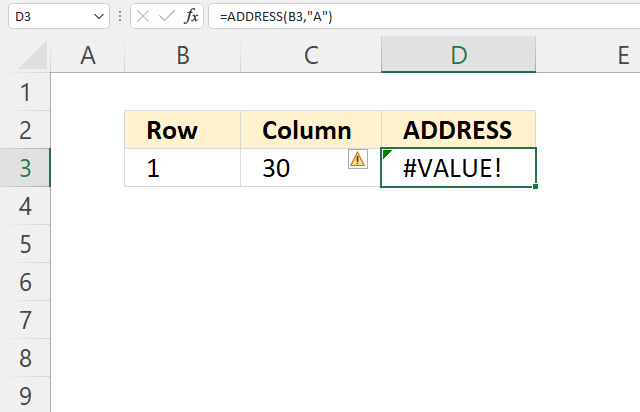The image above shows a #VALUE! error, the second argument expects a cell reference or a number, however, a text string is used.

## 5. Get value based on row and column number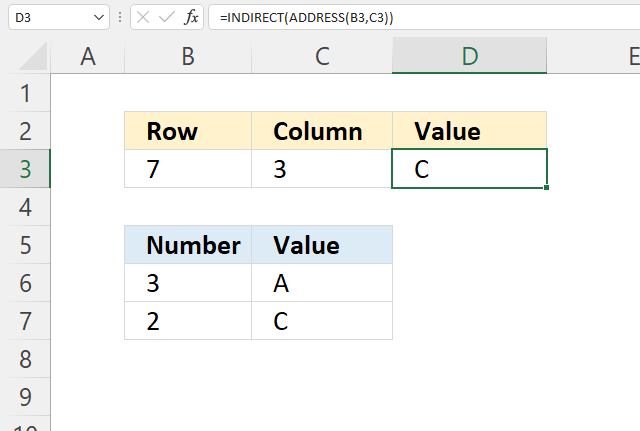Formula in cell D3:

### 5.1 Explaining formula

becomes

and returns C7.

#### Step 2 - Convert string to cell reference

The INDIRECT function creates a cell reference from on a value.

INDIRECT(ref_text, [a1])

becomes

INDIRECT("C7")

and returns "C".

The INDIRECT function is a volatile function meaning it will recalculate more often than non-volatile functions. Extensive use of the INDIRECT function or other volatile functions may slow down your workbook considerably.

## 6. Get value based on row and column number - ADDRESS function alternative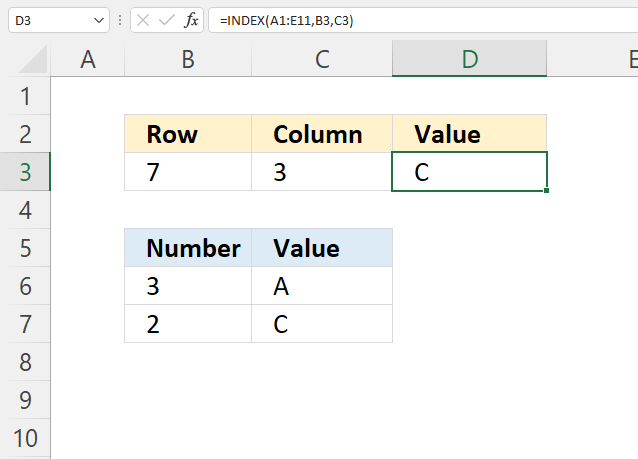I recommend the INDEX function instead of the ADDRESS function to create a cell reference that you will be using to get a value.

Formula in cell D3:

=INDEX(A1:E11,B3,C3)

### 6.1 Explaining formula

#### Step 1 - INDEX function

The INDEX function returns

INDEX(reference, [row_num], [column_num], [area_num])

reference -
row_num -
column_num -
area_num -

#### Step 2 - Populate arguments

INDEX(reference, [row_num], [column_num], [area_num])

reference - A1:E11
row_num - B3
column_num - C3
area_num - optional

#### Step 3 - Evaluate INDEx function

INDEX(A1:E11,B3,C3)

becomes

INDEX(A1:E11,7,3)

becomes cell reference C7

and returns "C".

## 7. Get value based on a row and column number and a worksheet name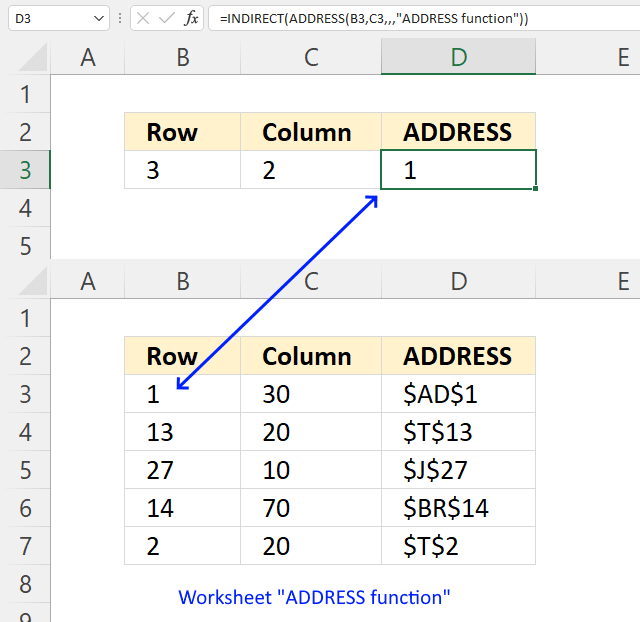The image above demonstrates a formula in cell D3 that returns a value from another sheet using the ADDRESS function.

Formula in cell D3:

### 7.1 Explaining formula

#### Step 1 - ADDRESS function

The ADDRESS function calculates the address of a specific cell based on a row and column number.

#### Step 2 - Populate arguments

row_num - B3

column_num - C3

[abs_num], - optional

[a1] - optional

becomes

#### Step 3 - Create cell reference

The INDIRECT function creates a cell reference from on a value.

INDIRECT(ref_text, [a1])

becomes

and returns 1.

## 8. Create an address to a cell range and return values from that cell range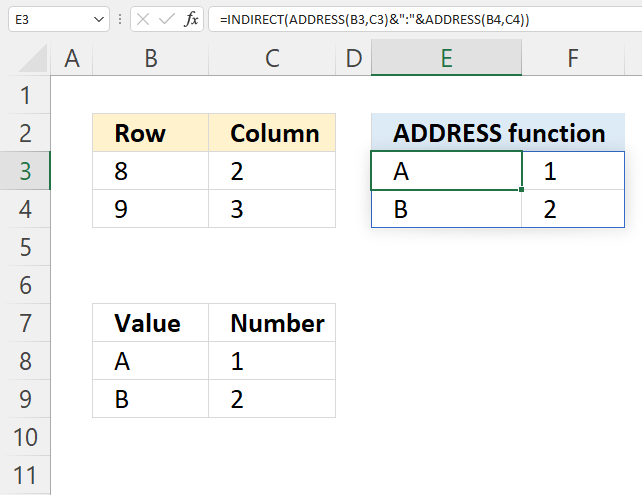The image above shows a formula in cell E3 that creates a cell reference to a cell range using the ADDRESS function.

Array formula in cell E3:

### 8.1 Explaining formula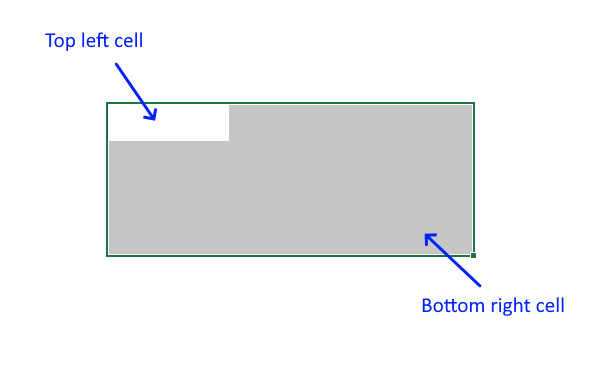#### Step 1 - Top left cell

becomes

and returns string "B8".

#### Step 2 - Bottom right cell

becomes

and returns string "C9".

#### Step 3 - Create a cell address to a cell range

The ampersand character lets you concatenate text strings in an Excel formula.

becomes

B8&":"&C9

and returns the string "B8:C9".

#### Step 4 - Create a cell reference

The INDIRECT function creates a cell reference from on a value.

INDIRECT(ref_text, [a1])

becomes

INDIRECT("B8:C9")

and returns cell reference B8:C9.

## 9. Extract column letter - Excel 365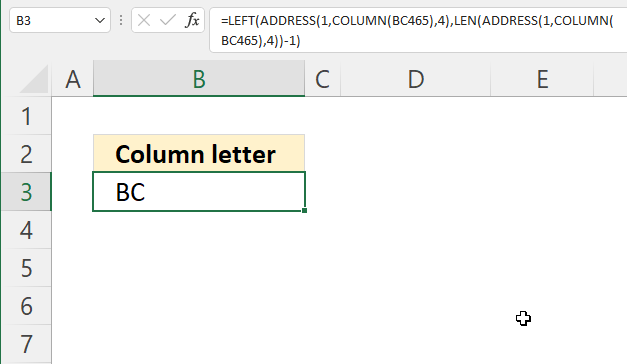The image above shows a formula in cell B3 that extracts the column letters from a given cell reference, in this example BC465.

Excel 365 formula in cell B3:

=LET(x, ADDRESS(1, COLUMN(BC465), 4), LEFT(x, LEN(x)-1))

### Explaining formula

#### Step 1 - Get column number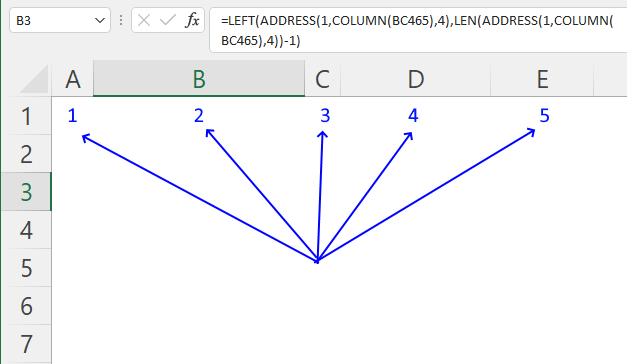The COLUMN function returns a number representing the column of a given cell reference counting from the left.

COLUMN(cell_ref)

COLUMN(BC465)

returns 55. Column BC465 is the 55th column counting from the left, see the image above.

#### Step 2 - Create cell address

[abs_num] - Optional. Let's you choose the type of reference to return.
1 - Absolute.
2 - Absolute row, relative column.
3 - Absolute column, relative row.
4 - Relative.

becomes

and returns BC1.

#### Step 3 - Count characters

The LEN function returns a number representing the total amount of characters in a string.

LEN(str)

becomes

LEN("BC1")-1

becomes

3 - 1 equals 2.

#### Step 4 - Remove row number

The LEFT function extracts a specific number of characters always starting from the left.

becomes

LEFT("BC1",2)

and returns "BC".

#### Step 5 - Shorten formula

The LET function lets you name intermediate calculation results which can shorten formulas considerably and improve performance.